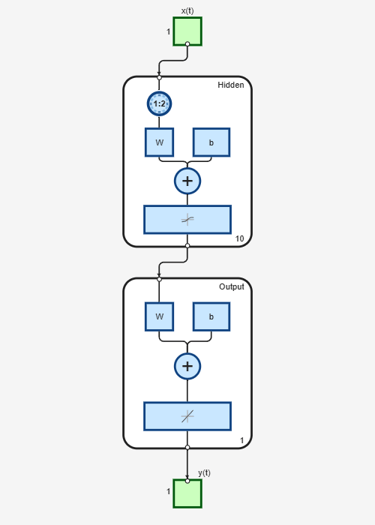# timedelaynet

Time delay neural network

## Syntax

```timedelaynet(inputDelays,hiddenSizes,trainFcn) ```

## Description

Time delay networks are similar to feedforward networks, except that the input weight has a tap delay line associated with it. This allows the network to have a finite dynamic response to time series input data. This network is also similar to the distributed delay neural network (`distdelaynet`), which has delays on the layer weights in addition to the input weight.

`timedelaynet(inputDelays,hiddenSizes,trainFcn)` takes these arguments,

 `inputDelays` Row vector of increasing 0 or positive delays (default = 1:2) `hiddenSizes` Row vector of one or more hidden layer sizes (default = 10) `trainFcn` Training function (default = `'trainlm'`)

and returns a time delay neural network.

## Examples

collapse all

Partition the training set. Use `Xnew` to do prediction in closed loop mode later.

```[X,T] = simpleseries_dataset; Xnew = X(81:100); X = X(1:80); T = T(1:80); ```

Train a time delay network, and simulate it on the first 80 observations.

```net = timedelaynet(1:2,10); [Xs,Xi,Ai,Ts] = preparets(net,X,T); net = train(net,Xs,Ts,Xi,Ai); view(net) ```Calculate the network performance.

```[Y,Xf,Af] = net(Xs,Xi,Ai); perf = perform(net,Ts,Y); ```

Run the prediction for 20 timesteps ahead in closed loop mode.

```[netc,Xic,Aic] = closeloop(net,Xf,Af); view(netc) ``````y2 = netc(Xnew,Xic,Aic); ```